# Modified Dietz ratio

The Modified Dietz ratio, sometimes also referred to as the modified internal rate of return (MIRR), is a metric that measures the historical performance of a portfolio when in- or outflows occur. The modified Dietz ratio is similar to the dollar-weighted return, but it has the advantage that we do not require a solver to find the exact rate of return. The modified internal rate of return is recommended by the Investment Performance Council (IPC) under their Global Investment Performance Standards (GIPS).

On this page, we provide the formula needed to calculate the modified Dietz. We also provide an example and implement the approach in Excel, which can be downloaded at the bottom of this page.

# Modified Dietz formula

The modified Dietz formula looks as follows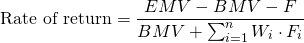where BMV is the beginning market value, EMV is the ending market value, F is the net external inflow of new funds for the period, and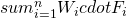is het sum of each flow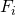multiplied by its weight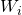.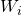is the fraction of the time between the point in time when the flowtakes place and the end of the period. Thus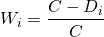where C is the total number of calendar days during the investment period being calculated, which equals end date minus start date, and Di is the number of days from the start of the investment period until the day on which Fi takes place.

## Simple Dietz Method

There is also a simple Dietz method, which is a special case of the above Modified Dietz method. In this case, the inflows are assumed to take place at the midpoint of every period. In the formula we provide above, the actual timing of every external flow is taken into consideration.

## Modified Dietz method example

Let’s consider a very simple example that illustrates how investors can calculate this ratio. Suppose we have a portfolio that is worth a 100 at the beginning of the investment period. At the end of the investment period (e.g. after two years), the portfolio value has increased to 250. At the end of the first year, there was also an inflow of 50.

First we need to calculate the profit or loss over the two-year period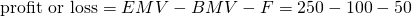or 50. Then, we have to calculate the average capital over the investment period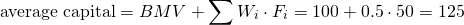Using the above results, we can calculate the Modified Dietz return as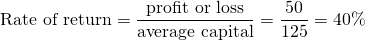## Summary

We have provided and applied the Modified Dietz ratio, sometimes also referred to as the modified irr (mirr). This measure is very important in the context of performance reporting and it is closely related to the dollar-weighted return.

### Modified Dietz calculator

Want to have a Modified Dietz calculator in Excel? Download the Excel file here: Modified Dietz.